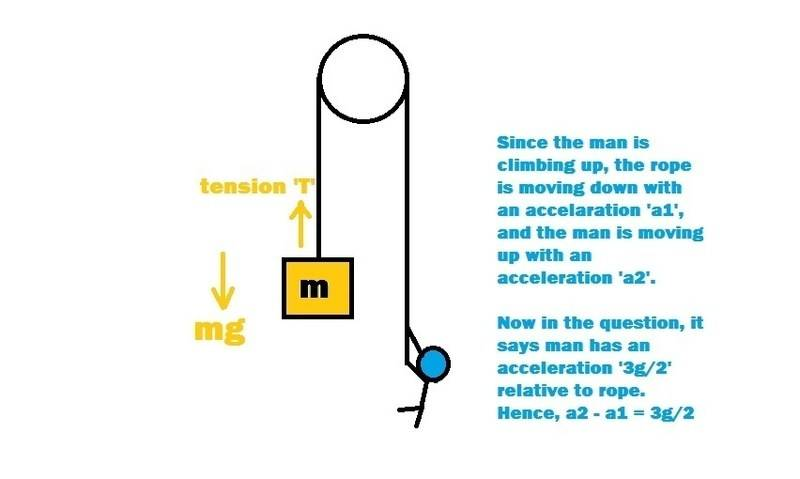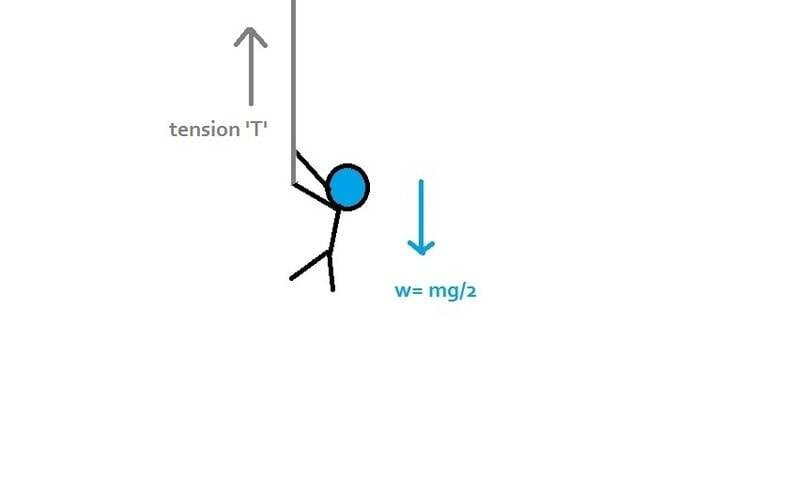# Find the tension in the rope

## Homework Statement

In order to raise a mass 'm' a man ties it to a rope and passes the rope over a friction less pulley. He climbs the rope with an acceleration 3g/2 relative to the rope. If the mass of the man is m/2 and the mass of the rope is negligible, the tension in the rope is
a) 3mg/2
b) 5mg/2
c)7mg/6
d) 9mg/7

F=ma

## The Attempt at a Solution

So basically we have a friction less pulley, and over which passes a rope. One end has been connected to the mass 'm' which, on drawing the free body diagram will show two forces, mg and the tension. While the other end, where the man is climbing the rope is getting very messy to approach with :/

TSny
Homework Helper
Gold Member
Welcome to PF!
While the other end, where the man is climbing the rope is getting very messy to approach with :/
In terms of dealing with the forces applied to the man, it is really not different than dealing with the forces on the mass m. Can you describe your attempt to draw a free body diagram for the man?

•ItsAnshumaan
Chestermiller
Mentor
Most pulley problems must start out with a consideration of the kinematics. If the mass is downward with an acceleration a (relative to the ground), what is the upward acceleration of the portion of the rope on the man's side of the pulley (relative to the ground)? If the man is moving upward relative to the rope on his side of the pulley with an acceleration 3g/2, in terms of g and a, what is the man's acceleration relative to the ground?

•ItsAnshumaan
Welcome to PF!

In terms of dealing with the forces applied to the man, it is really not different than dealing with the forces on the mass m. Can you describe your attempt to draw a free body diagram for the man?

OK here is what I tried to doSo I am not able to figure out what to do with the force which is helping the man to climb up.

Most pulley problems must start out with a consideration of the kinematics. If the mass is downward with an acceleration a (relative to the ground), what is the upward acceleration of the portion of the rope on the man's side of the pulley (relative to the ground)? If the man is moving upward relative to the rope on his side of the pulley with an acceleration 3g/2, in terms of g and a, what is the man's acceleration relative to the ground?

The mass is actually moving up, I can figure out that the only equation we can get out of that mass is: T - mg = m a1 (Here I am considering a1 as acceleration of the rope and a2 as the acceleration of the man relative to the rope).

So the man's acceleration with respect to ground must be; a2 = 3g/2 + a1

TSny
Homework Helper
Gold Member
What equation do you get from setting up Newton's second law for the man?

For the relation between a1 and a2, you will need to be careful with the signs. For instance, if the rope were accelerating downward on the right side of the pulley at 2 m/s2 and the man were accelerating upward at 3 m/s2 with respect to the ground, then what would be the value of the acceleration of the man with respect to the rope?

•ItsAnshumaan
What equation do you get from setting up Newton's second law for the man?

For the relation between a1 and a2, you will need to be careful with the signs. For instance, if the rope were accelerating downward on the right side of the pulley at 2 m/s2 and the man were accelerating upward at 3 m/s2 with respect to the ground, then what would be the value of the acceleration of the man with respect to the rope?

Yes, the value of the acceleration of the man with respect to the rope would be basically 1 m/s2 upwards.

Okay so with that knowledge, I can say that the man is moving up with an acceleration (3g/2 + a1) m/s2, and the overall sum of all the forces acting on the man would be equal to (m/2)(3g/2 + a1).

Now regarding the free body diagram of the man, I get 2 forces: 1) Weight(vertically downwards = mg/2) 2) And the other force I think must be the frictional force helping the man to move up.
I am not sure regarding the 2nd force, I am getting confused at that point. I don't exactly understand which force is responsible for the man to help climb the rope.

TSny
Homework Helper
Gold Member
Yes, the value of the acceleration of the man with respect to the rope would be basically 1 m/s2 upwards.
Actually, it would not be 1 m/s2. If the rope on the right side were accelerating upward at 2 m/s2 with respect to the ground while the man accelerated upward at 3 m/s2 with respect to the ground, then the acceleration of the man with respect to rope would be 1 m/s2. But what if the rope on the right side accelerates downward at 2 m/s2 with respect to the ground while the man accelerates upward at 3 m/s2 with respect to the ground?

Okay so with that knowledge, I can say that the man is moving up with an acceleration (3g/2 + a1) m/s2, and the overall sum of all the forces acting on the man would be equal to (m/2)(3g/2 + a1).
This is not correct if a1 represents the downward acceleration of the rope on the right side.

Now regarding the free body diagram of the man, I get 2 forces: 1) Weight(vertically downwards = mg/2) 2) And the other force I think must be the frictional force helping the man to move up.
I am not sure regarding the 2nd force, I am getting confused at that point. I don't exactly understand which force is responsible for the man to help climb the rope.
Yes, the upward force on the man is the friction force from the rope and the downward force on the man is the weight of the man. Can you see a relation between the friction force and the tension in the rope?

Last edited:
•ItsAnshumaan
Actually, it would not be 1 m/s2. If the rope on the right side were accelerating upward at 2 m/s2 with respect to the ground while the man accelerated upward at 3 m/s2 with respect to the ground, then the acceleration of the man with respect to rope would be 1 m/s2. But what if the rope on the right side is accelerating downward at 2 m/s2 with respect to the ground while the man accelerated upward at 3 m/s2 with respect to the ground?
Oh right finally understood, 5m/s2 upwards.

So then, 3g/2 = a1 + a2, that means acceleration of the man is; a2 = (3g/2 - a1).

Then for the man, the relation would be: f - mg = (m/2)(3g/2 - a1). Is this correct now? (f is the frictional force)

TSny
Homework Helper
Gold Member
Oh right finally understood, 5m/s2 upwards.

So then, 3g/2 = a1 + a2, that means acceleration of the man is; a2 = (3g/2 - a1).

Then for the man, the relation would be: f - mg = (m/2)(3g/2 - a1). Is this correct now? (f is the frictional force)
Yes. That all looks good. Just need to figure out how f is related to T.
Edit: I just noticed that you don't have the correct mass on the left side of your last equation above.

•ItsAnshumaan
Yes. That all looks good. Just need to figure out how f is related to T.
Edit: I just noticed that you don't have the correct mass on the left side of your last equation above.

Sorry that would be f - mg/2 = (m/2)(3g/2 - a1).

But I am unable to draw the relation between f and T..

TSny
Homework Helper
Gold Member
If the rope pulls upward on the man with the force f, then the man pulls down on the rope with the force f. Consider the section of rope that experiences the downward force f from the man. The net force on this section must be zero (since the rope is massless and a nonzero net force would cause the section to have infinite acceleration). So, some other force must act on this section of rope.

•ItsAnshumaan
Oh yes, so that force must be the tension. In that case T = f, or T = mg/2 + m/2(3g/2 + m a1)

So the earlier equation i had for the block was, T - mg = m a1.. So a1 = (T - mg)/m

Putting the value of a1 in T = mg/2 + m/2(3g/2 + m a1), I am very unfortunate to get the value T = {mg(4-m) / (2-m)}, as there is no such option from the multiple given answers.. Any idea where I went wrong?

Oh yes, so that force must be the tension. In that case T = f, or T = mg/2 + m/2(3g/2 + m a1)

So the earlier equation i had for the block was, T - mg = m a1.. So a1 = (T - mg)/m

Putting the value of a1 in T = mg/2 + m/2(3g/2 + m a1), I am very unfortunate to get the value T = {mg(4-m) / (2-m)}, as there is no such option from the multiple given answers.. Any idea where I went wrong?
## T = \frac{mg}{2} + \frac{m}{2} ( \frac{3g}{2} + m a_1) ##
First, Why is there ## m a_1 ## ? Why do you have the mass of the object in there?
Second thing is, You already have an equation for the acceleration of the man to something stationary (Ground) and it is ## a_2 = \frac{3g}{2} - a_1 ##

Try now and see if it gives you the correct answer

•ItsAnshumaan
## T = \frac{mg}{2} + \frac{m}{2} ( \frac{3g}{2} + m a_1) ##
First, Why is there ## m a_1 ## ? Why do you have the mass of the object in there?
Second thing is, You already have an equation for the acceleration of the man to something stationary (Ground) and it is ## a_2 = \frac{3g}{2} - a_1 ##

Try now and see if it gives you the correct answer

Thanks a lot! Turns out that I had copied the equation wrong. Yes it worked, and the answer I got is 7mg/6, perfectly the 3rd option! :)

Thanks Chestermiller, TSny and Biker for your help, now I am able understand the pulley problems to the core!

Thanks a lot! Turns out that I had copied the equation wrong. Yes it worked, and the answer I got is 7mg/6, perfectly the 3rd option! :)
No problem at all, Welcome to Physics forums

Thanks Chestermiller, TSny and Biker for your help, now I am able understand the pulley problems to the core!